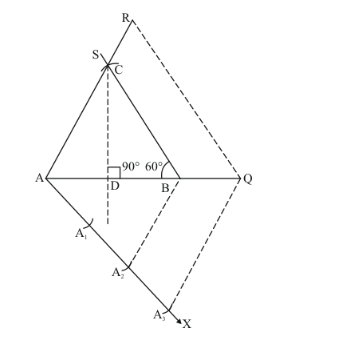# Construct a ΔABC in which AB = 5 cm. ∠B = 60° altitude CD = 3 cm

Question:

Construct a ΔABC in which AB = 5 cm. ∠B = 60° altitude CD = 3 cm. Construct a ΔAQR similar to ΔABC such that side ΔAQR is 1.5 times that of the corresponding sides of ΔACB

Solution:

Given that

Construct a triangle $\triangle A B C$ in which $\operatorname{let} A B=5 \mathrm{~cm}, \angle B=90^{\circ}$ and altitutde $C D=3 \mathrm{~cm}$, and then a triangle $\triangle A Q R$ similar to it whose sides are $(1.5$ times $=3 / 2)$ of the corresponding sides of $\triangle A C B$.

We follow the following steps to construct the givenStep of construction

Step: I- First of all we draw a line segment $A B=5 \mathrm{~cm}$, .

Step: Il- With $B$ as centre and draw an angle $\angle B=60^{\circ}$.

Step: III -From point $A$ and $B$ construct altitutde $C D=3 \mathrm{~cm}$, which cut the line $B S$ at point $C$

Step: IV- Join $A C$ to obtain $\triangle A B C$.

Step: V-Below $A B$, makes an acute angle $\angle B A X=60^{\circ}$.

Step: VI- Along $A X$, mark off five points $A_{1}, A_{2}$ and $\mathrm{A}_{3}$ such that $A A_{1}=A_{1} A_{2}=A_{2} A_{3}$

Step: VII -Join $A_{2} B$.

Step: VIII - Since we have to construct a triangle $\triangle A Q R$ each of whose sides is $(1.5$ times $=3 / 2)$ of the corresponding sides of $\triangle A B C$.

So, we draw a line $A_{3} Q$ on $A X$ from point $A_{3}$ which is $A_{3} Q \| A_{2} B$, and meeting $A B$ at $Q$.

Step: IX- From $Q$ point draw $Q R \mid B C$, and meeting $A C$ at $R$

Thus, $\triangle A Q R$ is the required triangle, each of whose sides is $(1.5$ times $=3 / 2)$ of the corresponding sides of $\triangle A B C$.